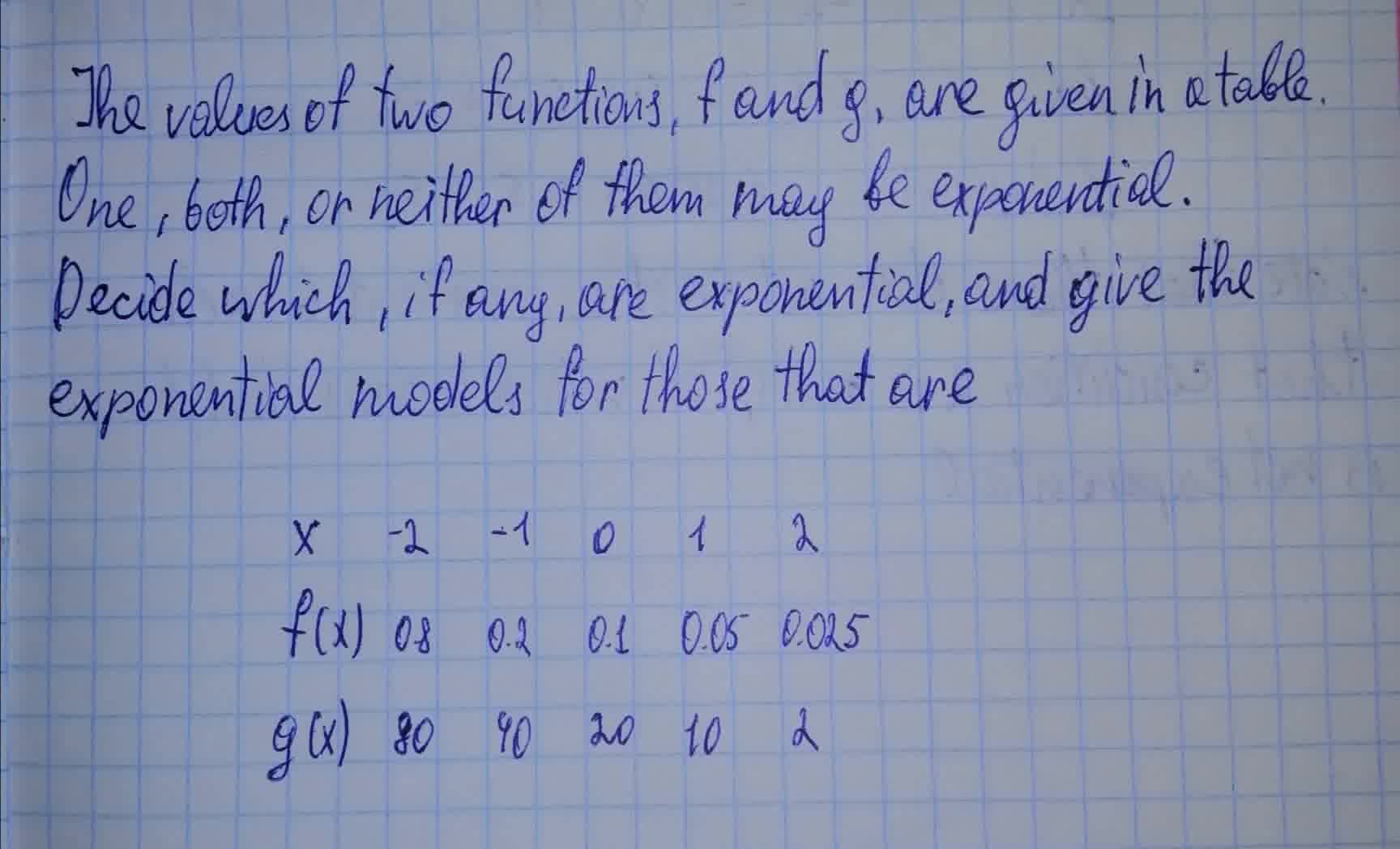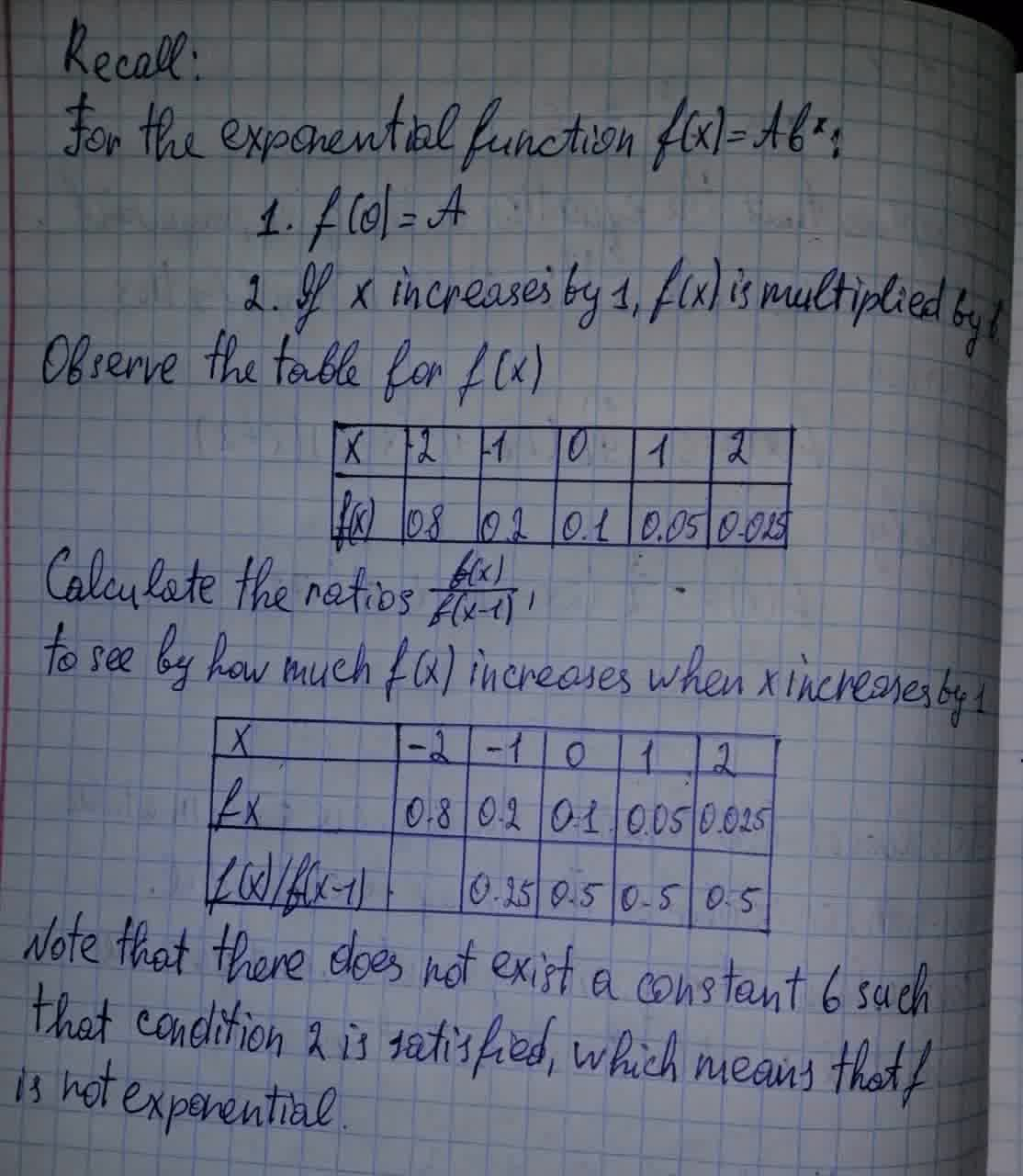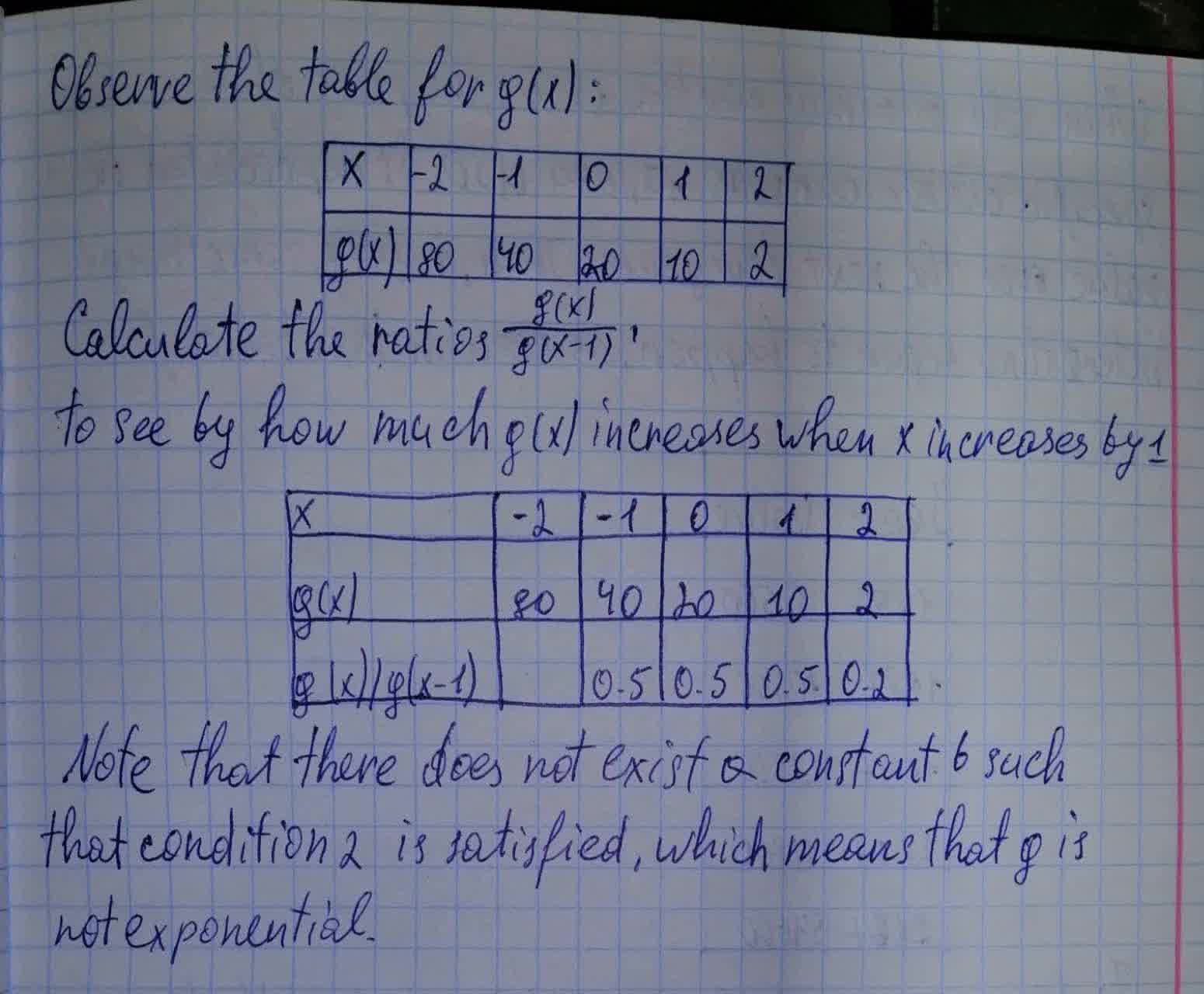FizeauV

2021-07-20

The values of two functions, f and g, are given in a table. One, both, or neither of them may be exponential. Decide which, if any, are exponential, and give the exponential models for those that are.
$\begin{array}{}x& -2& -1& 0& 1& 2\\ f\left(x\right)& 0.8& 0.2& 0.1& 0.005& 0.025\\ g\left(x\right)& 80& 40& 20& 10& 2\end{array}$Dora

Given that:Do you have a similar question?